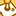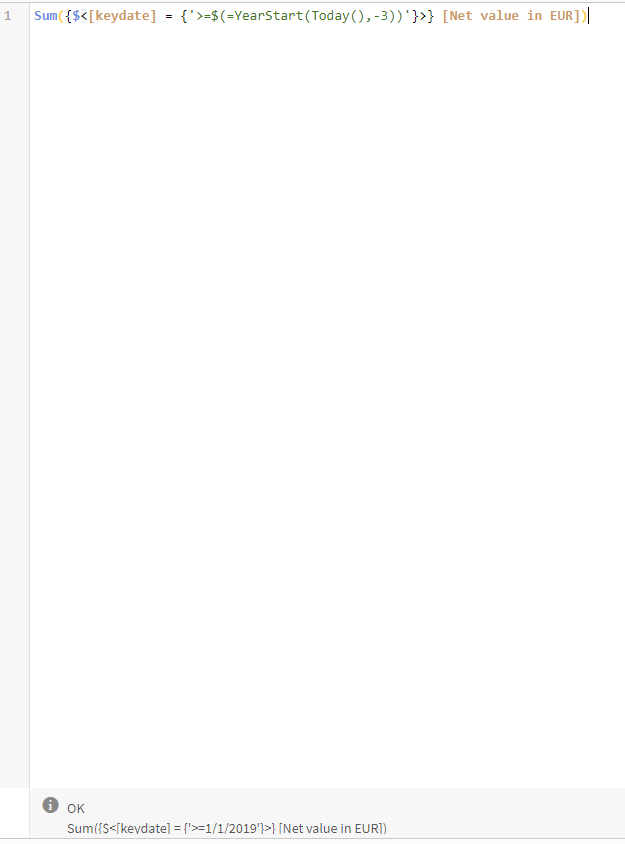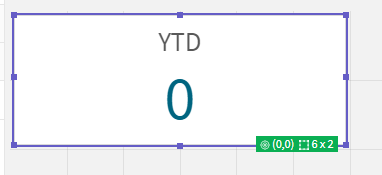# New to Qlik Sense

If you’re new to Qlik Sense, start with this Discussion Board and get up-to-speed quickly.

cancel
Showing results for
Did you mean:Not applicable

## Set Analysis - YTD Compared with Previous Years (Same Parameter)

Hi All,

I am using Qlik Sense and I am wanting to create a set analysis function that gives me the ability to display dynamic Year on Year variances using a Year To Date function.

For example, I want to retrieve total sales up to now for 2016 (YTD). I then want to compare this figure with the exact same time parameter but in the previous years.

At the moment I am using expressions like the following:

This basic expression retrieves latest YTD figure

SUM({\$<Year_Calendar={\$(=max(Year_Calendar))}>}Sales)

I can use the following function to retrieve the previous year, however as there is nothing limiting the calculation to today's date, it returns the figure for the whole of the previous year (Which I cannot use to make valid YoY comparisons).

SUM({\$<Year_Calendar={\$(=max(Year_Calendar)-1)}>}Sales)

I am therefore thinking I should use an expression that limits to today's date also, something like this... (not sure if this will work)

SUM({\$<Year_Calendar={">=\$(=MAX(Year_Calendar))<\$(=MAX(CalDate))"}>}Sales)  ??

But how would I then apply this for previous years?

In simple terms, the outcome I want is:

To calculate total sales between 01/01/2016 - 24/08/2016 (YTD),

then calculate total sales between 01/01/2015 - 24/08/2015 (YTD - 1Year),

Then compare.

Any advice would be much appreciated, thanks.

Lewis

1 Solution

Accepted SolutionsNot applicable
Author

This works well

YTD

Sum({\$<[Date] = {'>=\$(=YearStart(Today()))'}>} [Sales])

YTD Previous Year

YTD YoY Variance

(Sum({\$<[Date] = {'>=\$(=YearStart(Today()))'}>} [Sales]) - Sum({\$<[Date] = '>=\$(=Date(AddYears(YearStart(Today()),-1)))<\$(=Date(AddYears(Today(),-1)))'}>} [Sales]))

3 RepliesMVP

Sum({<Year_Calendar, CalDate = {"\$(='>=' & Date(YearStart(Max(CalDate), -1), 'DD/MM/YYYY') & '<' & Date(AddYears(Max(CalDate), -1), 'DD/MM/YYYY'))"}>}Sales)Not applicable
Author

This works well

YTD

Sum({\$<[Date] = {'>=\$(=YearStart(Today()))'}>} [Sales])

YTD Previous Year

YTD YoY Variance

(Sum({\$<[Date] = {'>=\$(=YearStart(Today()))'}>} [Sales]) - Sum({\$<[Date] = '>=\$(=Date(AddYears(YearStart(Today()),-1)))<\$(=Date(AddYears(Today(),-1)))'}>} [Sales]))Contributor

Hello everybody,

YTD

Sum({\$<[Date] = {'>=\$(=YearStart(Today()))'}>} [Sales])

YTD Previous Year

YTD YoY Variance

(Sum({\$<[Date] = {'>=\$(=YearStart(Today()))'}>} [Sales]) - Sum({\$<[Date] = '>=\$(=Date(AddYears(YearStart(Today()),-1)))<\$(=Date(AddYears(Today(),-1)))'}>} [Sales]))

I tried this solution in my Case but it doesn't work for my case.What informations do you need to  help me to solve it please ?Tags
Community Browser